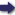(London :  Kegan Paul, Trench, Trübner & Co.,  1910.)

 Tools

## Search this bookPrev Page 57 Next``` ( 57 ) CHAPTEE LIV. ON THE COMPUTATION OF THE MEAN PLACES OF THE PLANETS. If we know the number of cycles of the planets in a General kalpct or caturyuga, and further know how many cycles the deter- have elapsed at a certain moment of time, we also the mean know that the sum-total of the days of the kalpct or planet at ccduryuga stands in the same relation to the sum-total ti^e^^^ " of the cycles as the past days of the kalpa or cctturyugct to the corresponding amount of planetary cycles. The most generally used method is this :— The past days of the kalpa or caturyuga are multi¬ plied by the cycles of the planet, or of its apsis, or of its node which it describes in a kalpct or caturyugct. The product is divided by the sum-total of the days of the kctlpa or cctturyuga accordingly as you reckon by the one or the other. The quotient represents complete cycles. These, however, because not wanted, are dis¬ regarded. The remainder which you get by the division is mul¬ tiplied by 12, and the product is divided by the sum- total of the days of either kalpa or caturyuga by which we have already once divided. The quotient repre¬ sents signs of the ecliptic. The remainder of this divi¬ sion is multiplied by 30, and the product divided by the same divisor. The quotient represents degrees. The remainder of this division is multiplied by 60, and is divided by the same divisor. The quotient represents minutes. ```Prev Page 57 Next